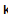Projection of a Vector onto a Plane - Maple Help

Home : Support : Online Help : Math Apps : Algebra and Geometry : Points, Lines, and Planes : Projection of a Vector onto a Plane

Projection of a Vector onto a Plane

 Main Concept Recall that the vector projection of a vector  onto another vector  is given by .   The projection of  onto a plane can be calculated by subtracting the component of   that is orthogonal to the plane from  .  If you think of the plane as being horizontal, this means computing   minus the vertical component of , leaving the horizontal component. This "vertical" component is calculated as the projection of   onto the plane normal vector .

Choose the coordinates of a plane normal vector $\stackrel{\mathit{⇀}}{n}$ and a vector  and notice how the perpendicular of the vector projection of  onto $\stackrel{\mathit{⇀}}{n}$ is the projection of  onto the plane.Normal Vector $\stackrel{{\mathbf{⇀}}}{{\mathbit{n}}}$ Vector $\stackrel{{\mathbf{⇀}}}{{\mathbit{u}}}$${x}_{u}=$${y}_{n}=$${y}_{u}=$${z}_{n}=$${z}_{u}=$More MathApps